Published © CC BY-NC-SA

# Word Clock!

Are you tired of looking at spinning hands or plain numbers? This clock gives you the time in English!

IntermediateShowcase (no instructions)3,327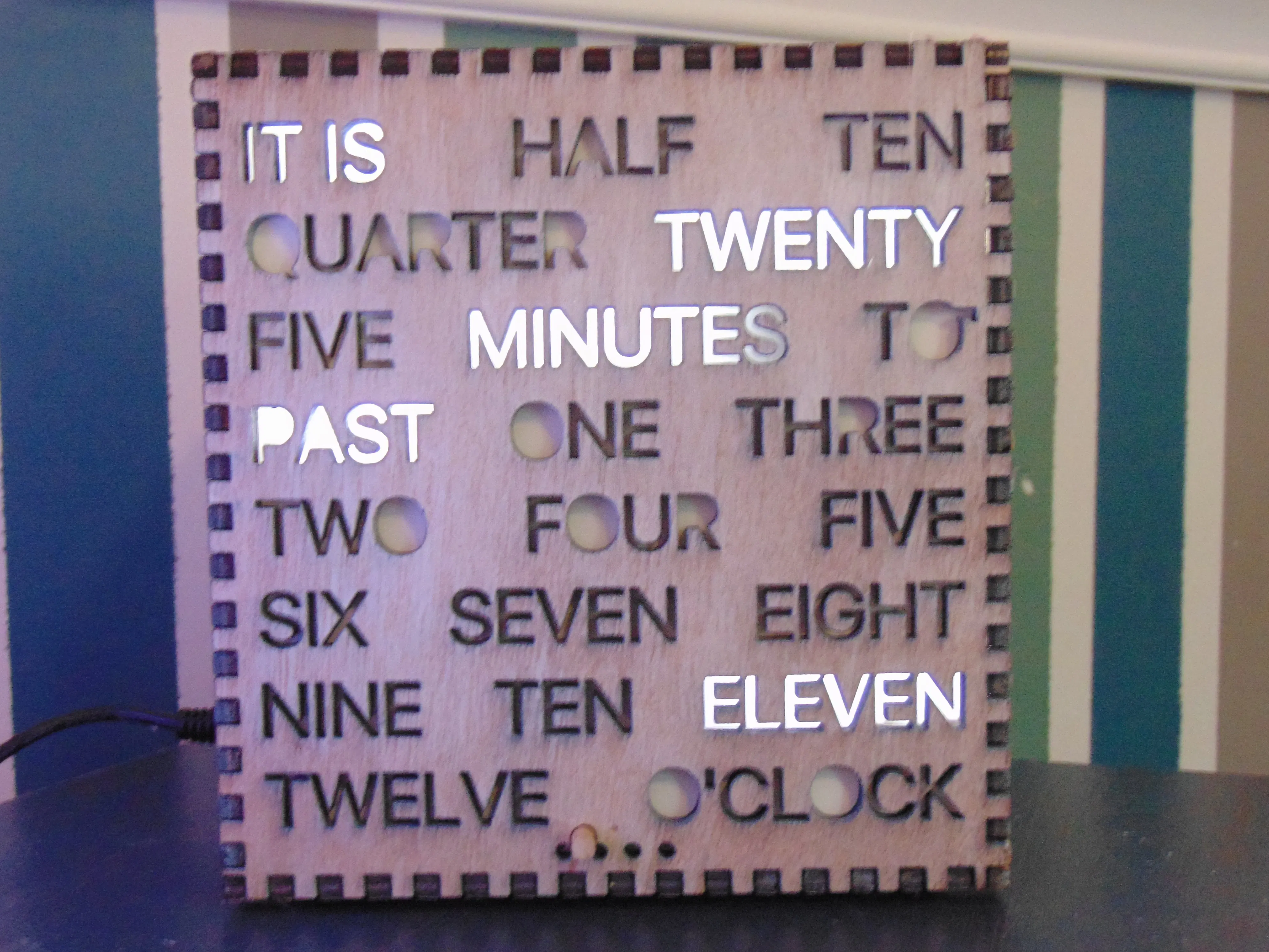## Things used in this project

### Hardware components

 Adafruit DS1307 RTC Breakout
×1
 Adafruit 24 Channel PWM LED Driver
×1
 Adafruit SD card to SPI adaptor
×1Arduino UNO
×1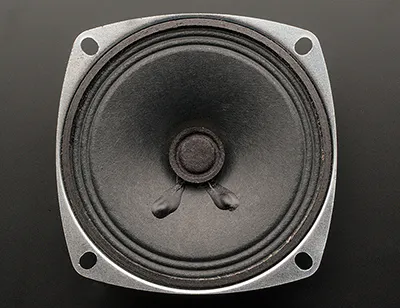Speaker: 3W, 4 ohms
×1
 IRF540n N Channel Mosfet
×1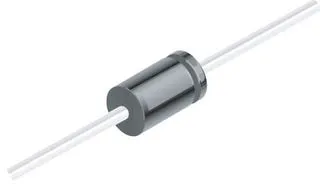1N4007 – High Voltage, High Current Rated Diode
×1Resistor 10k ohm
×2
 Micro Switch/Button
×1
 Micro SD Card Any size
×1Jumper wires (generic)
×1

### Hand tools and fabrication machinesSoldering iron (generic)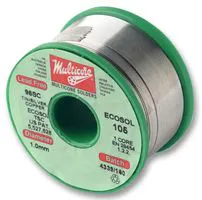Solder Wire, Lead FreeWire Stripper & Cutter, 18-10 AWG / 0.75-4mm² Capacity Wires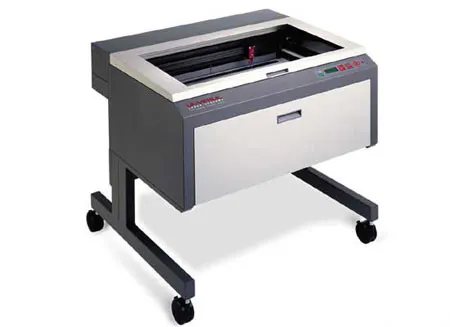Laser cutter (generic)

Read more

## Custom parts and enclosures

### Case

This is meant to be cut on a k40 laser. Bigger bed sizes will also work. The main parts are for 5 mm plywood, and the filter is for anything thin and semi transparent. It is optional to have the filter

## Schematics

### The Schematic

Connect all the things like so.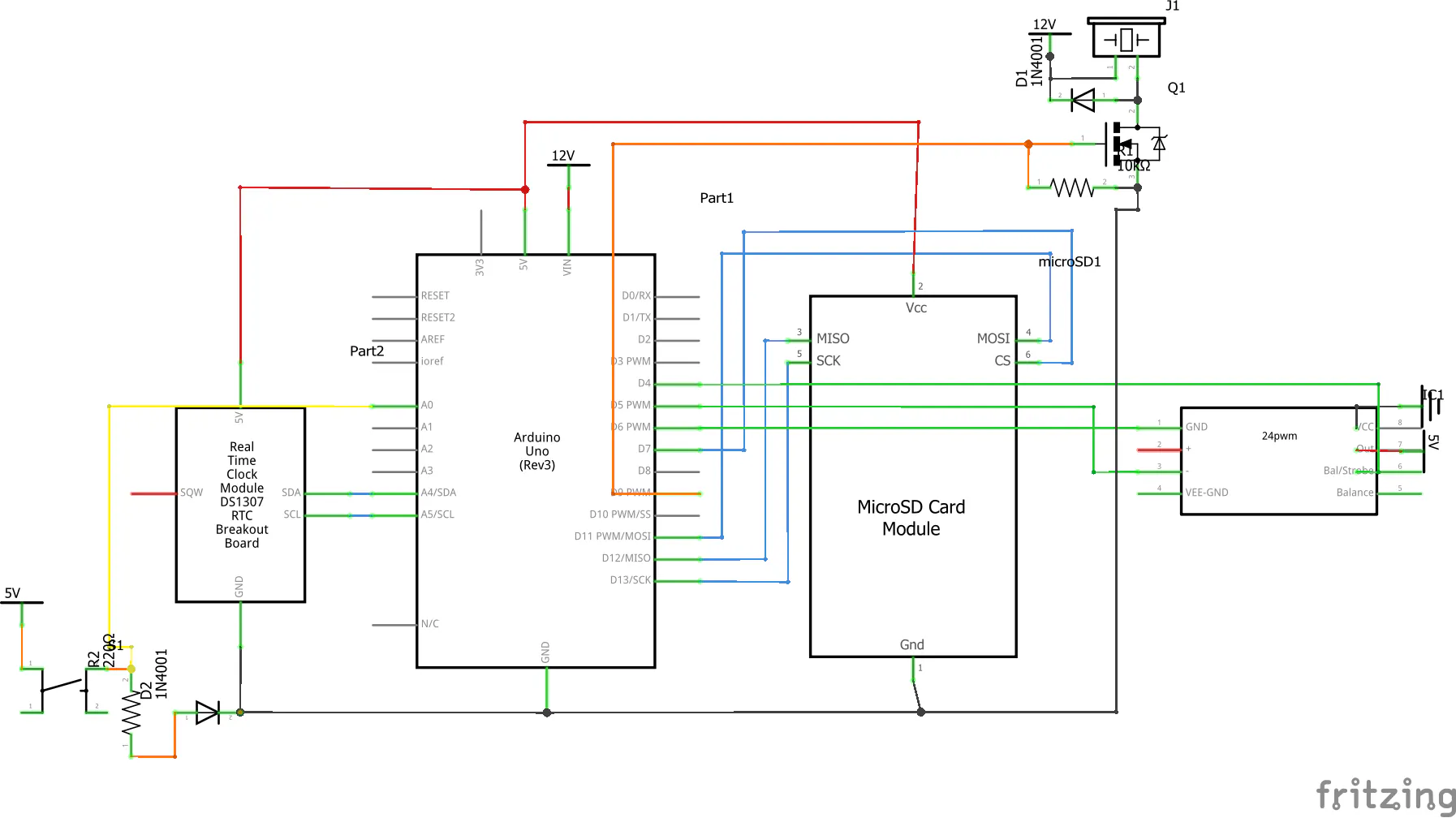## Code

### The Code! Version 2

C/C++
Paste, Upload, Enjoy.
To anyone who saw this message, the bug is fixed!
```//Look though this code if you want. Anything with a comment is changeable.

#include <Adafruit_TLC5947.h>
#include <Wire.h>
#include <RTClib.h>
#include <SD.h>
#include <TMRpcm.h>
#include <SPI.h>

#define SD_ChipSelectPin 8
#define NUM_TLC5974 1
#define din 4
#define clock 5
#define latch 6
RTC_DS1307 rtc;
TMRpcm tmrpcm;
Adafruit_TLC5947 tlc = Adafruit_TLC5947(NUM_TLC5974, clock, din, latch);

//Alarm Times
byte alarmhours = 8;
byte alarmminutes = 0;

String inputString = "";
boolean stringComplete = false;
int t = 0;
int hou = 0;
int m = 0;
int s = 0;
String data = "";
bool buttonpress = false;
int light = 4096;
byte to = 0;
byte past = 0;
bool itis = false;
bool mins = false;
byte h = 0;
int button = 0;
bool alarmran = false;

void setup () {
rtc.begin();
inputString.reserve(200);

tmrpcm.speakerPin = 9;
if (!SD.begin(SD_ChipSelectPin))
{
Serial.println("SD fail");
return;
}
//Speaker Volume 1-6 1=quiet 6=loudest + audio cliping. 5 is best
tmrpcm.setVolume(5);

Serial.begin(57600);
if (! rtc.begin()) {
Serial.println("Couldn't find RTC");
while (1);
}
if (! rtc.isrunning()) {
Serial.println("RTC is NOT running!");
rtc.adjust(DateTime(2019, 6, 11, 0, 0, 0));
}

tlc.begin();
}

void displayupdate() {
DateTime now = rtc.now();

for (uint16_t i = 0; i < 8; i++) {
tlc.setLED(i, 0, 0, 0);
}

past = 0;
to = 0;

itis = false;

if (now.minute() > 25 && now.minute() <= 30) {
tlc.setLED(0, light, light, 0);
itis = true;
}
if (now.minute() > 5 && now.minute() <= 10) {
tlc.setLED(0, light, 0, light);
itis = true;
}
if (now.minute() > 45 && now.minute() <= 50) {
tlc.setLED(0, light, 0, light);
itis = true;
}

if (itis == false) {
tlc.setLED(0, light, 0, 0);
}

if (now.minute() > 10 && now.minute() <= 15) {
tlc.setLED(1, light, 0, 0);
}
if (now.minute() > 40 && now.minute() <= 45) {
tlc.setLED(1, light, 0, 0);
}
if (now.minute() > 15 && now.minute() <= 20) {
tlc.setLED(1, 0, light, 0);
}
if (now.minute() > 35 && now.minute() <= 40) {
tlc.setLED(1, 0, light, 0);
}
if (now.minute() > 0 && now.minute() <= 5) {
tlc.setLED(1, 0, 0, light);
}
if (now.minute() > 50 && now.minute() <= 55) {
tlc.setLED(1, 0, 0, light);
}
if (now.minute() > 20 && now.minute() <= 25) {
tlc.setLED(1, 0, light, light);
}
if (now.minute() > 30 && now.minute() <= 35) {
tlc.setLED(1, 0, light, light);
}

if (now.minute() > 30 && now.minute() < 55) {
to = 1;
}
if (now.minute() < 31 && now.minute() > 0) {
past = 1;
}

mins = false;
if (now.minute() > 5 && now.minute() <= 10) {
tlc.setLED(2, light, light * to, light * past);
mins = true;
}
if (now.minute() > 45 && now.minute() <= 50) {
tlc.setLED(2, light, light * to, light * past);
mins = true;
}
if (now.minute() > 15 && now.minute() <= 25) {
tlc.setLED(2, light, light * to, light * past);
mins = true;
}
if (now.minute() > 30 && now.minute() <= 40) {
tlc.setLED(2, light, light * to, light * past);
mins = true;
}
if (now.minute() > 50 && now.minute() <= 55) {
tlc.setLED(2, light, light * to, light * past);
mins = true;
}
if (now.minute() > 0 && now.minute() <= 5) {
tlc.setLED(2, light, light * to, light * past);
mins = true;
}

if (mins == false) {
tlc.setLED(2, 0, light * to, light * past);
}

if (now.minute() > 30 && now.minute() < 55) {
h = now.hour() + 1;
}
if (now.minute() <= 30) {
h = now.hour();
}

if (h == 1) {
tlc.setLED(3, light, 0, 0);
}
if (h == 2) {
tlc.setLED(3, 0, 0, light);
}
if (h == 3) {
tlc.setLED(3, 0, light, 0);
}
if (h == 4) {
tlc.setLED(4, light, 0, 0);
}
if (h == 5) {
tlc.setLED(4, 0, light, 0);
}
if (h == 6) {
tlc.setLED(4, 0, 0, light);
}
if (h == 7) {
tlc.setLED(5, light, 0, 0);
}
if (h == 8) {
tlc.setLED(5, 0, light, 0);
}
if (h == 9) {
tlc.setLED(5, 0, 0, light);
}
if (h == 10) {
tlc.setLED(6, light, 0, 0);
}
if (h == 11) {
tlc.setLED(6, 0, light, 0);
}
if (h == 12) {
tlc.setLED(6, 0, 0, light);
}

if (h == 13) {
tlc.setLED(3, light, 0, 0);
}
if (h == 14) {
tlc.setLED(3, 0, 0, light);
}
if (h == 15) {
tlc.setLED(3, 0, light, 0);
}
if (h == 16) {
tlc.setLED(4, light, 0, 0);
}
if (h == 17) {
tlc.setLED(4, 0, light, 0);
}
if (h == 18) {
tlc.setLED(4, 0, 0, light);
}
if (h == 19) {
tlc.setLED(5, light, 0, 0);
}
if (h == 20) {
tlc.setLED(5, 0, light, 0);
}
if (h == 21) {
tlc.setLED(5, 0, 0, light);
}
if (h == 22) {
tlc.setLED(6, light, 0, 0);
}
if (h == 23) {
tlc.setLED(6, 0, light, 0);
}
if (h == 0) {
tlc.setLED(6, 0, 0, light);
}

if (now.minute() == 0 or now.minute() > 55) {
tlc.setLED(7, light, 0, 0);
}

tlc.write();
}

void addition() {
DateTime now = rtc.now();
// Dims Light
if (now.hour() < 6 or now.hour() > 21) {//Change the times for on/off light dimming
light = 500; //Dimmest 0-4096
}
else {
light = 4096;// Brightest
}
}

void(* resetFunc) (void) = 0;

void loop () {
//Set time from Serial Monitor. Enter each number hours,minutes,second press enter in between
serialEvent();
displayupdate();
addition();

if (stringComplete) {
Serial.println(h);
t = inputString.toInt();
if (hou != 0 && m != 0 && s == 0) {
s = t;
}
if (hou != 0 && m == 0) {
m = t;
}
if (hou == 0) {
hou = t;
}
if (hou != 0 && m != 0 && s != 0) {
rtc.adjust(DateTime(2000, 0, 0, hou, m, s));
hou = 0;
m = 0;
s = 0;
}
inputString = "";
stringComplete = false;
}

//Alarm
DateTime now = rtc.now();
if (alarmhours == now.hour() && alarmminutes == now.minute() && alarmran == false) {
tmrpcm.play("test.wav");
do {
button = analogRead(0);
if (button > 800) {
buttonpress = false;

}
else {
buttonpress = true;
tmrpcm.disable();
}
} while (tmrpcm.isPlaying() == 1 && buttonpress == false);
alarmran = true;
tmrpcm.disable();
}

if (now.hour() == 12 && now.minute() == 1 && now.second() == 15) {
//alarmran = false;
delay(1000);
resetFunc();
}
if (now.hour() == 1 && now.minute() == 1 && now.second() == 15) {
//alarmran = false;
delay(1000);
resetFunc();
}

delay(1000);
}

void serialEvent() {
while (Serial.available()) {
char inChar = (char)Serial.read();
inputString += inChar;
if (inChar == '\n') {
stringComplete = true;
}
}
}
```

## Credits

### NoraTheDoggo

0 projects • 2 followers